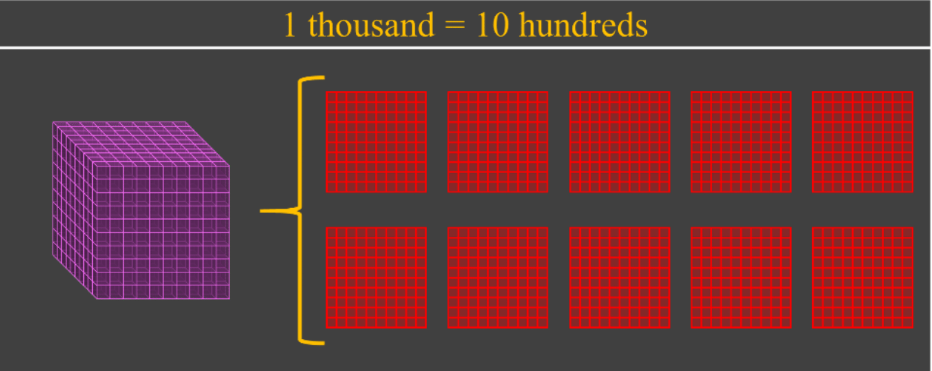# Subtracting with regrouping (using base ten blocks)#### Everything You Need in One Place

Homework problems? Exam preparation? Trying to grasp a concept or just brushing up the basics? Our extensive help & practice library have got you covered.#### Learn and Practice With Ease

Our proven video lessons ease you through problems quickly, and you get tonnes of friendly practice on questions that trip students up on tests and finals.#### Instant and Unlimited Help

Our personalized learning platform enables you to instantly find the exact walkthrough to your specific type of question. Activate unlimited help now!

0/2
##### Intros
###### Lessons
1. Introduction to Subtracting with Regrouping (using base ten block models):
2. How to use base ten blocks to model numbers
3. How to show regrouping using block models for subtraction
0/6
##### Examples
###### Lessons
1. Subtracting 2-digit numbers without regrouping (using base ten block models)
Subtract:
1. 16 - 4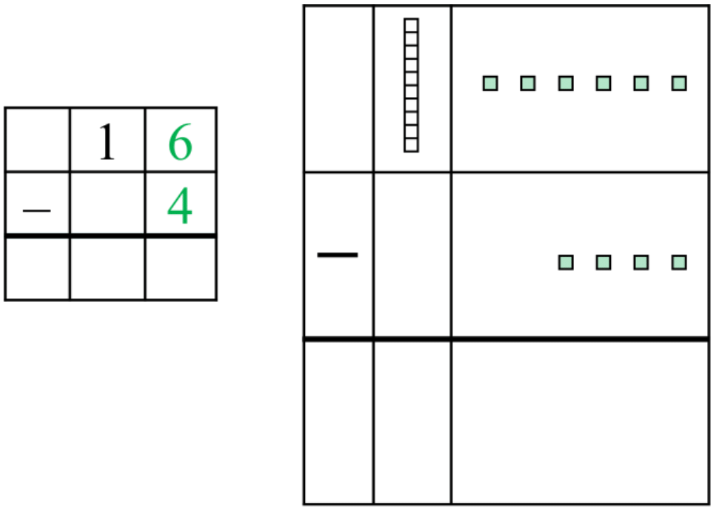2. 38 - 15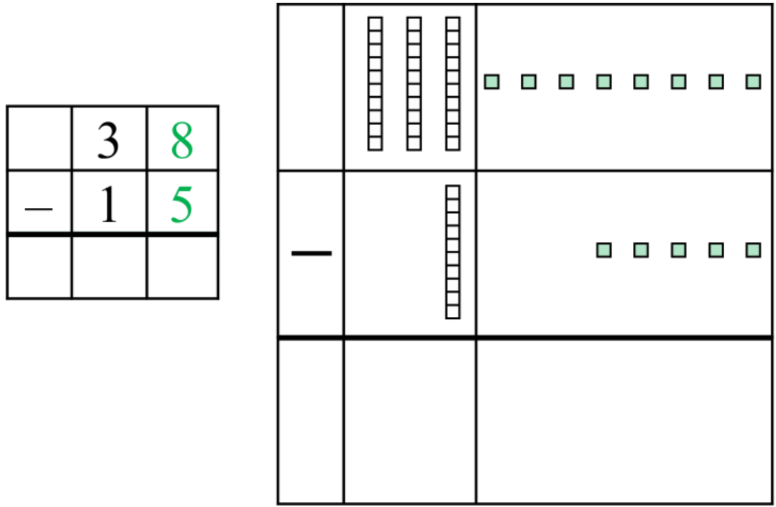2. Subtracting 2-digit numbers with regrouping (using base ten blocks)
Subtract:

1.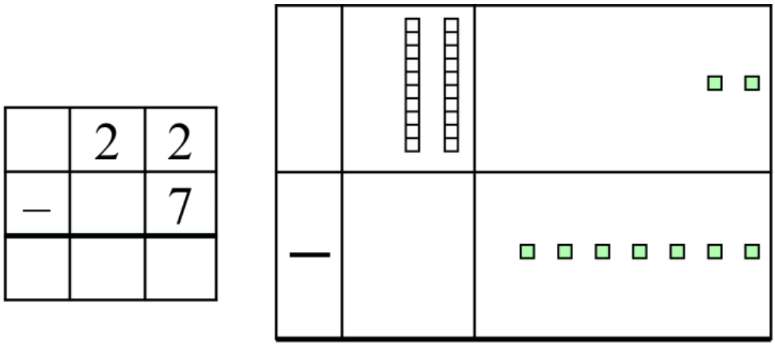2.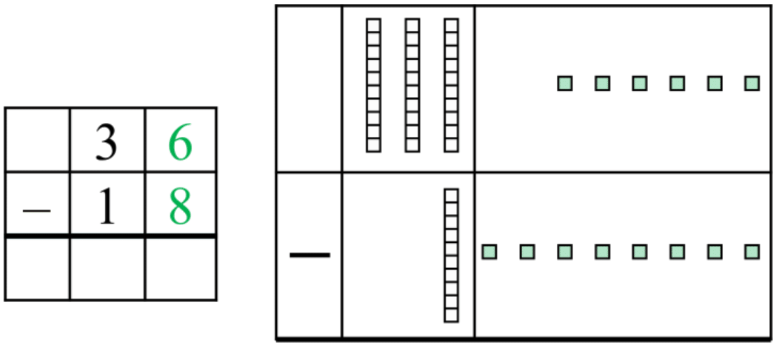3. Subtracting 3-digit numbers with regrouping (using base ten blocks)
Subtract: 468 - 281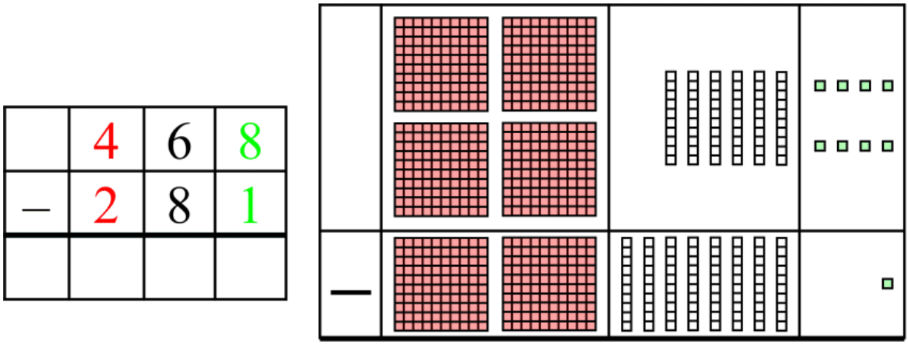1. Word problem for subtracting with regrouping (with base ten block models)
On Halloween, Freddy had 159 candies to give out. After the first two hours, he had already given out 73 candies. In the next two hours, he gave out 61 more candies. How many candies does Freddy have left at the end of the night?

###### Topic Notes

In this lesson, we will learn:

• How to regroup base ten block models ("borrowing" or "exchanging")
• How to subtract using base ten block models

Notes:

• Like addition, we will also need to regroup our base ten blocks for subtraction

• When we don't have enough to subtract, we need to borrow from the next place value up to make more (splitting up the base ten block models)
• Sometimes, this is called "exchanging"

1 ten can be exchanged into 10 ones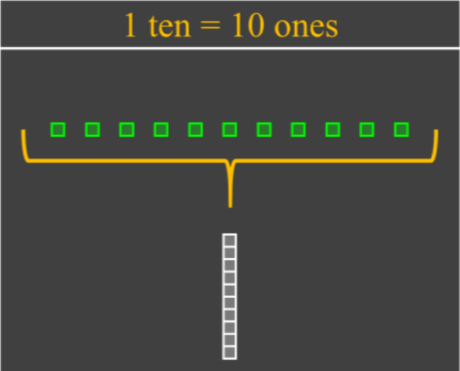1 hundred can be exchanged into 10 tens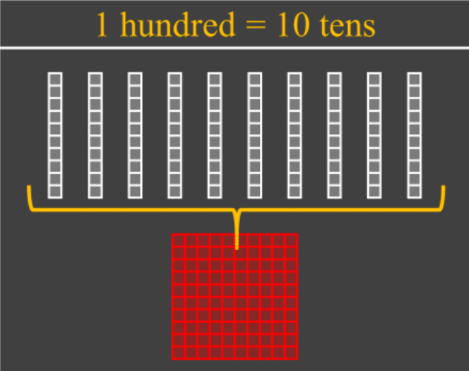1 thousand can be exchanged into 10 hundreds